From 9:00 AM - 12:00 PM CDT on Saturday, June 6th, ni.com will be undergoing system upgrades that may result in temporary service interruption.

We appreciate your patience as we improve our online experience.

## LabVIEW 2018 Help

Edition Date: March 2018
Part Number: 371361R-01
View Product InfoLabVIEW 2016 HelpLabVIEW 2017 HelpLabVIEW 2018 HelpLabVIEW 2019 HelpLabVIEW 2020 Help

Owning Palette: Scaling VIs

Requires: Base Development System

Converts a thermistor voltage into a temperature. This VI has different modes of operation for voltage-excited and current-excited thermistors. Wire data to the Voltage input to determine the polymorphic instance to use or manually select the instance.

Use the pull-down menu to select an instance of this VI.Type of Excitation distinguishes the two modes of operation of the VI. This VI defaults to the Voltage Reference mode. Use the Voltage Reference (VREF) and the R1 values only in this mode. You use the Current Excitation (IEX) value only in the Current Reference mode. This input accepts the following values.

 0 Voltage Reference 1 Current Reference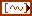Voltage is the voltage read from a thermistor.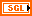Voltage Reference (VREF) is the reference you apply across a resistor of known value in series with your thermistor. The default is 2.5 V. Use this input only when you set Type of Excitation to Voltage Reference.R1 is the value of the resistor in series with your thermistor expressed in Ohms. The default is 5 kΩ. Use this input only when you set Type of Excitation to Voltage Reference.Excitation Current (IEX) is the current excitation applied to the thermistor. The default is 100 µA. Use this input only when you set Type of Excitation to Current Reference.Temperature Units is the units of temperature the VI outputs. This input accepts the following values.

 0 Celsius 1 Fahrenheit 2 Kelvin 3 Rankine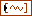Temperature is the return temperature value in the units specified in Temperature Units.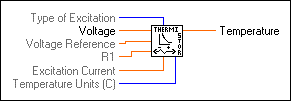Type of Excitation distinguishes the two modes of operation of the VI. This VI defaults to the Voltage Reference mode. Use the Voltage Reference (VREF) and the R1 values only in this mode. You use the Current Excitation (IEX) value only in the Current Reference mode. This input accepts the following values.

 0 Voltage Reference 1 Current ReferenceVoltage is the voltage read from a thermistor.Voltage Reference (VREF) is the reference you apply across a resistor of known value in series with your thermistor. The default is 2.5 V. Use this input only when you set Type of Excitation to Voltage Reference.R1 is the value of the resistor in series with your thermistor expressed in Ohms. The default is 5 kΩ. Use this input only when you set Type of Excitation to Voltage Reference.Excitation Current (IEX) is the current excitation applied to the thermistor. The default is 100 µA. Use this input only when you set Type of Excitation to Current Reference.Temperature Units is the units of temperature the VI outputs. This input accepts the following values.

 0 Celsius 1 Fahrenheit 2 Kelvin 3 RankineTemperature is the return temperature value in the units specified in Temperature Units.

The Convert Thermistor Reading VI has two modes of operation for use with different types of thermistor circuits. The following illustration shows how the thermistor can be connected to a voltage reference.The following illustration shows a circuit where the thermistor is excited by a constant current source.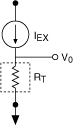If the thermistor is excited by voltage, the following equation shows the relationship of the thermistor resistance, RT, to the input values.If the thermistor is excited by current, the following equation shows the relationship of the thermistor resistance, RT, to the input values.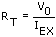The following equation shows the standard formula the VI uses for converting a thermistor resistance to temperature.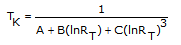The values used by this VI for A, B, and C are given below. If you are using a thermistor with different values for A, B, and C (refer to the thermistor data sheet), you can edit the VI diagram to use different A, B, and C values.

A = 1.295361E-3

B = 2.343159E-4

C = 1.018703E-7

The VI produces a temperature in degrees Celsius. Therefore, TC = TK – 273.15.